## Tuesday, June 22, 2010

### Examples of Self-Similar Tile Sets (Part 3)

These are examples of tilings from a variety of polygons. My purpose for making these is art, not math. These tilings, or tile patches, are one solution to a problem I encountered while generating tilings as structures for a series of vertex pattern diagrams. I needed a way to create greater density changes than I was getting with other tilings. Self-similar tile sets solve the problem. See Part 1 and Part 2 for detailed descriptions of two of these examples.

The tile set I describe in Part 1 is closely related to, and can be derived from tilings described by Robert W. Fathauer. Fathauer found two families of self-similar tilings based on segments of regular polygons [See the references below]. He went on to discover a great variety of fractal tilings that, as tilings, are more interesting than most of those I describe here. Unlike Fathauer’s discoveries the tilings below are either trivial, or they require more than one prototile. My method for developing a tiling is not necessarily straight forward or fixed. My purpose is not to generate interesting fractal diagrams, but to develop often asymmetrical structures for vertex pattern diagrams.

I've imposed a few restrictions on these tilings. That is, they should be edge-to-edge, with no overlaps. Tilings may not fill the plane, but should be infinitely scalable at the boundaries, as in a fractal. In some examples there might be gaps, but these gaps might be bounded by infinitely scaleable tiles forming singularities. These are actually tile patches, not tessellations. In most of these examples the tile set could be extended by adding other polygons, especially triangles.

1: A square and a triangle. The triangle is the s=4 case described by Fathauer, and I have retained the square for which the triangle is a segment. In most of these examples I'm showing one possible tiling that might extend indefinitely in a similar way.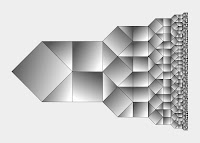2: A pentagon and a triangle. The triangle is the s=10 case described by Fathauer, and I have retained the pentagon that remains after removing five s=10 tiles from a regular decagon. This is the tile set I describe in Part 1.3: A regular hexagon and two triangles. The isosceles triangle subdivides the equilateral triangle, which subdivides the hexagon. Therefore, a similar tiling could be accomplished with just an isosceles triangle.4: Two trapezoids and two triangles. Other tilings could be made with just the two trapezoids or one trapezoid and a triangle.5: A dart and a triangle. In this example the triangle is necessary to continue the scaling when the darts fold in on themselves. Darts alone without the triangles would overlap.6: Another dart. Unlike the previous example, no triangle is necessary. When the darts fold in on themselves the gap can be bounded by infinitely scalable darts.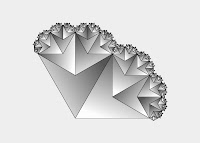7: Another dart and triangle. A right isosceles triangle or square is necessary to fill a gap when the darts fold in on themselves.8. A hexagon. This example is trivial, but the hexagon prototile can be combined with triangles that subdivide the hexagon to create interesting asymmetries. The hexagon fits inside a regular pentagon. This is the tile set I describe in Part 2.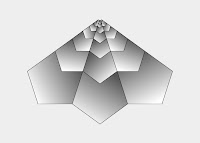9: A pentagon and two triangles. The pentagon fits inside a regular hexagon.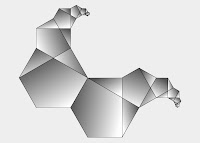10: Golden Rectangles. The lengths of the two longest sides of each L-shaped hexagon are in the golden ratio.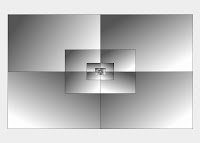References

Fathauer, Robert W. (2000). "Self-similar Tilings Based on Prototiles Constructed from Segments of Regular Polygons," presented at the Bridges Conference (July 28-30, 2000, Southwestern College, Winfield, Kansas).
http://www.mathartfun.com/shopsite_sc/store/html/Compendium/Bridges2000.pdf
Also see:
http://www.mathartfun.com/shopsite_sc/store/html/Compendium/encyclopedia.html